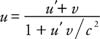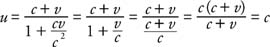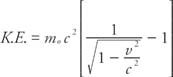## Relativity

Albert Einstein devised the special theory of relativity to reconcile discrepancies between the fields of electromagnetism and mechanics. Special relativity is the mechanics of objects moving at high velocities, near the speed of light, in contrast to Newtonian mechanics, which deals with velocities found in daily life. Einstein is quoted as saying, “Common sense is that layer of prejudice laid down prior to the age of sixteen.” This remark was prompted by the realization that the laws of special relativity are contrary to our common sense because we do not travel at speeds near the speed of light.

General relativity treats gravitational fields as equivalent to acceleration. The theory relates to the physics of the stars and even to the evolution of the universe—cosmology.

## Frames of reference

Newton's laws apply in any nonaccelerating frame of reference, called an inertial frame, that is, one with constant velocity. According to Newtonian physics, if the laws of mechanics are valid in one inertial frame of reference, they must be valid in any inertial frame of reference. For example, if the laws of mechanics are valid in an experiment on a train moving with a constant velocity, they must also be valid at the railroad station. Imagine that a person on the train throws a ball straight up. A person observing this action from the railroad platform will insist that the ball had a parabolic path due to the forward motion of the train. Each, however, will agree that the ball obeys the law of mechanics. Furthermore, the person at the station may say that he is at rest while the train moves past in one direction; the person on the train may say that she is at rest and that the station is moving past in the opposite direction. As long as the frame of reference is nonaccelerating, there is no way to prove that any given frame of reference is absolutely at rest, nor is there a preferred frame of reference.

## Michelson-Morley experiment

The statement that the laws of mechanics are valid in all frames of reference led to a contradiction when applied to Maxwell's electromagnetic equations. Maxwell predicted that the speed of light always propagated through a vacuum with a unique speed c. Classical mechanics could not explain why light should always travel with the same speed, regardless of the frame of reference.

In the nineteenth century, scientists believed that all waves must travel on some medium; thus, light was said to travel on ether, sometimes called luminous ether (not at all the same thing as the chemical compound). The ether had very unusual properties: Scientists said it had to exist in glass, other transparent materials, and even a vacuum because light travels through them; it had to be exceptionally thin because there was no discernible drag on planets in orbit; and it had to be undetectable and rigid in order to vibrate at the high frequencies of light waves. If light traveled with a constant speed with respect to a specific frame of reference, it was thought it might be that of the ether—the absolute frame.

Albert A. Michelson (1852–1931) and Edward W. Morley (1838–1923) used an interferometer to investigate the ether. A beam of light was split into two paths. One path was parallel to the direction of the motion of the earth, and the other path was perpendicular to the first. The earth's moving through the ether would be equivalent to an ether wind blowing in the opposite direction, which was parallel to the interferometer. The interference of the recombination of the two beams at the telescope gave an interference pattern of fringes. Then the interferometer was rotated through 90 degrees to see if the velocity of light changed with direction. The fringe shift was not observed. The null result was interpreted in two ways: (1) Ether does not exist; therefore, the electromagnetic wave does not need a medium for its propagation. (2) There is no preferred frame of reference—no absolute frame of reference.

## The special theory of relativity

Einstein suggested that absolute motion has no meaning, that all motion is relative. He formulated two basic postulates for the special theory of relativity.

• The laws of physics are the same in all inertial reference frames.
• The speed of light is the same regardless of the frame of reference of the observer.
These simple postulates led to profoundly different ways of viewing the universe.

The first law seems reasonable; the second law is counterintuitive. In our experience, velocities simply add. For example, if a person throws a ball toward you at 10 m/s while riding a bicycle towards you at 10m/s, the ball seems to come at you at 20 m/s. The expression would be u = uv, where u is the velocity with respect to the ground, u′ is the velocity of the ball with respect to the bicycle, and v is the velocity of the bicycle.

The second law of special relativity leads to a different scenario for light. If a person rides toward you at 90 percent the speed of light and turns on a flashlight, you would find the light coming toward you at c, the speed of light. Moreover, this is the same speed observed if the bicycle were stationary relative to your position.

The different results in adding velocities from experience and from experiments with light beams could be explained only by assuming that adding low velocities is a special case of a more general law for the addition of velocities. Fizeau experimentally determined the correct equation for adding velocities to beThis equation yields the following equation for the speed of a light beam from a rider coming toward you with a speed of v:This equation for adding velocities gives the correct results as predicted by the second postulate of special relativity. Try other values to see that the combination of velocities less than C, and even equal to C, cannot give a combined value greater than C. Notice that if u and v are relatively small, the denominator becomes 1; then the expression reduces to the familiar equation for the addition of velocities.

## Time dilation and the Lorentz contraction

The consequence of Einstein's postulates is that the measurements of time and distance are not constant when compared from one inertial frame of reference to another so that the speed of light can remain a constant. In other words, there is no such thing as absolute time and absolute distance. All clocks in the universe do not keep time together. Time dilation is the effect that a moving clock runs slower than an identical stationary clock. The expression isFurthermore, not only does the clock run slower but so also does any physical process affected by the passing of time such as chemical and biological processes. Therefore, to the person in the moving frame, no change in time interval can be detected because all methods of measuring time are slowed by the same factor. All is relative.

Time dilation has been measured in a number of experiments. One experiment involved the change of time for decay of radioactive particles (muons) traveling to the surface of the earth at nearly the speed of light. The number of muons were counted at the top and at the bottom of a mountain. More muons were detected at the bottom than would be expected by classical theory. The time to decay was longer, and the value was consistent with the theory of special relativity. Accurate atomic clocks carried on airplanes and in orbiting satellites have all borne out the theory.

The Lorentz contraction is the effect that an observer moving with an object of a given length will find the object to be shortened compared to an observer at rest relative to this motion. (Note that this shortening occurs only in the direction of motion.) Again, all is relative. Because all distances in the direction of motion are shortened for the moving observer, there is no comparison length for the traveling observer to use to detect the change. The equation isTo connect the concept of length contraction to time dilation, imagine that you are on a muon coming to earth at nearly the speed of light. An observer on the ground could say it is now time for the particle to decay, but you could reply that by your clock the time has not yet arrived. Alternatively, if you calculate the height of the mountain, you will find it shortened in your frame of reference. In a sense, both time and distance are doctored up so that the speed of light is always the same.

Imagine one twin in a rocket ship traveling toward a star and returning at nearly the speed of light. The other twin stays home. The stay‐at‐home twin insists that she stayed at rest and the other twin moved. The twin in the rocket ship insists that she was at rest while the earth zoomed away from her and then returned.

The contradiction is that each can claim that the other moved and, therefore, was the one who aged less. Who did age the most? (It is not possible to have relative gray hair.)

To resolve the paradox, realize that the problem is not symmetric. When the rocket ship twin was leaving the earth, slowing to a stop in outer space, turning around to come home, and slowing to a stop at the earth's surface, she accelerated and decelerated; therefore, her frame of reference was not an inertial frame of reference. The traveling twin aged less.

## Relativistic momentum

The definition of momentum and energy must be generalized to fit within special relativity. The correct expression for relativistic momentum isNote that when v is low, the denominator is nearly 1, so momentum approaches the familiar equation: P = mv. This equation can be interpreted to mean that the mass is velocity‐dependent:When v is zero, the mass equals m 0. The term m 0 is called the rest mass.

## Relativistic energy

Einstein proposed the famous mass‐energy equivalence equation: E = mc 2. This energy is the sum of the kinetic and rest energies. The relationship shows that mass is a form of energy; therefore, a statement of conservation of energy must include the concept of mass. Because c is such a large value, a small mass is equivalent to an enormous energy.

The kinetic energy of a mass isBy using the binomial theorem to expand the expression, it can be shown that for small v this expression simplifies to the familiar## General relativity

The general theory of relativity is a theoretical framework applicable to any frame of reference—inertial or accelerating. In developing this theory, Einstein wanted to produce a theory of gravitation that incorporated the theory of special relativity and the equivalence principle.

To understand the equivalence principle, imagine that you are in a spaceship moving through outer space and cannot see outside the craft. After freely floating inside the craft, you start to drift to one end. Soon, you can stand on one wall. Either of two conditions could be in effect: (1) the spaceship is accelerating so that you are forced against the wall opposite the direction of acceleration, or (2) the ship has come close to a large mass with a gravitational field. The equivalence principle is that experiments conducted in either a uniformly accelerating frame of reference or in an inertial frame of reference with a gravitational field give the same results.

Einstein determined that the concepts of space should be reexamined. Newton's laws presuppose a Euclidean space extending in all directions, like three mutually perpendicular straight axes. Einstein proposed a curved space time. The motion of an object could be described in terms of the geometry of space, instead of as a response to applied forces.

For example, light travels in straight lines in Euclidean space; however, the general theory of relativity views space as distorted by mass. The light, therefore, might travel a curved path near a massive object, which is the shortest path between two points in that space. This is analogous to a curved path on the earth's surface, which is a shorter distance between two cities than a straight line between them.

To test his theories, Einstein proposed three phenomena that could be explained by the mathematical formulations of the general theory of relativity:

• The point of closest approach—the perihelion—of the elliptical orbit of Mercury advances about the sun. (Figure illustrates an exaggerated view of this motion.)
• The deflection of starlight passing near the sun is due to the warping of space near the solar mass.
• The gravitational redshift of light is a frequency shift between two identical clocks at different heights in a gravitational field.Figure 1 Advance of the perihelion of Mercury.

When Einstein suggested these tests, only the first phenomenon had been observed. Subsequently, scientists found and measured the other effects. All of the experimental data is consistent with the theory of general relativity.